#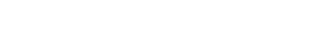Machine learning is commonly separated into three main learning paradigms: supervised learning, unsupervised learning, and reinforcement learning. These paradigms differ in the tasks they can solve and in how the data is presented to the computer. Usually, the task and the data directly determine which paradigm should be used (and in most cases, it is supervised learning). In some cases though, there is a choice to make. Often, these paradigms can be used together in order to obtain better results. This chapter gives an overview of what these learning paradigms are and what they can be used for.

### Supervised Learning

Supervised learning is the most common learning paradigm. In supervised learning, the computer learns from a set of input-output pairs, which are called labeled examples: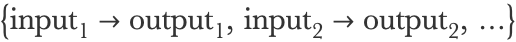The goal of supervised learning is usually to train a predictive model from these pairs. A predictive model is a program that is able to guess the output value (a.k.a. label) for a new unseen input. In a nutshell, the computer learns to predict using examples of correct predictions. For example, let’s consider a dataset of animal characteristics (note that typical datasets are much larger):

Our goal is to predict the weight of an animal from its other characteristics, so we rewrite this dataset as a set of input-output pairs:

The input variables (here, age and sex) are generally called features, and the set of features representing an example is called a feature vector. From this dataset, we can learn a predictor in a supervised way using the function Predict:

Now we can use this predictor to guess the weight of a new animal:

This is an example of a regression task (see Chapter 4, Regression) because the output is numeric. Here is another supervised learning example where the input is text and the output is a categorical variable ("cat" or "dog"):

Again, we can use the resulting model to make a prediction:

Because the output is categorical, this is an example of a classification task (see Chapter 3, Classification). The image identification example from the first chapter is another example of classification since the data consists of labeled examples such as:

As we can see, supervised learning is separated into two phases: a learning phase during which a model is produced and a prediction phase during which the model is used. The learning phase is called the training phase because the model is trained to perform the task. The prediction phase is called the evaluation phase or inference phase because the output is inferred (i.e. deduced) from the input.

Regression and classification are the main tasks of supervised learning, but this paradigm goes beyond these tasks. For example, object detection is an application of supervised learning for which the output consists of multiple classes and their corresponding box positions: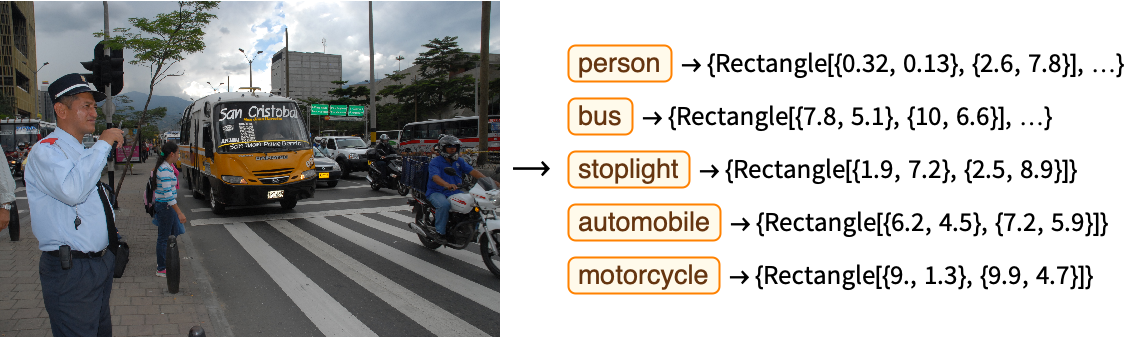Text translation and speech recognition, for which the output is text, are also tackled in a supervised way:

We could imagine all sorts of other output types. As long as the training data consists of a set of input-output pairs, it is a supervised learning task.

Most of the applications that we showed in the first chapter are learned in a supervised way. Currently, the majority of machine learning applications that are developed are using a supervised learning approach. One reason for that is that the main supervised tasks (classification and regression) are useful and well defined and can often be tackled using simple algorithms. Another reason is that many tools have been developed for this paradigm. The main downside of supervised learning, though, is that we need to have labeled data, which can be hard to obtain in some cases.

### Unsupervised Learning

Unsupervised learning is the second most used learning paradigm. It is not used as much as supervised learning, but it unlocks different types of applications. In unsupervised learning, there are neither inputs nor outputs, the data is just a set of examples: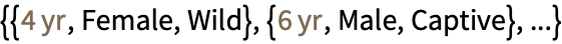Unsupervised learning can be used for a diverse range of tasks. One of them is called clustering (see Chapter 6, Clustering), and its goal is to separate data examples into groups called clusters: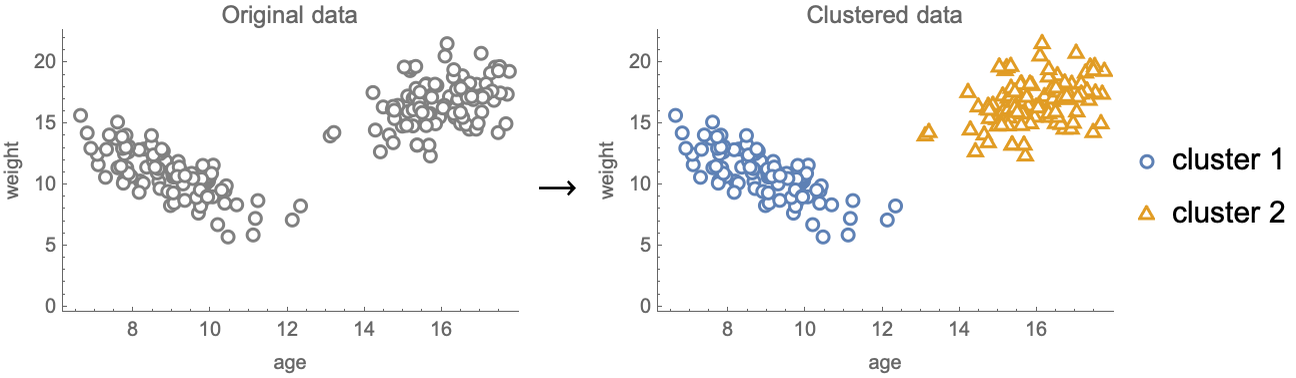An application of clustering could be to automatically separate customers of a company to create better marketing campaigns. Clustering is also simply used as an exploration tool to obtain insights about the data and make informed decisions.

Another classic unsupervised task is called dimensionality reduction (see Chapter 7, Dimensionality Reduction). The goal of dimensionality reduction is to reduce the number of variables in a dataset while trying to preserve some properties of the data, such as distances between examples. Here is an example of a dataset of three variables reduced to two variables: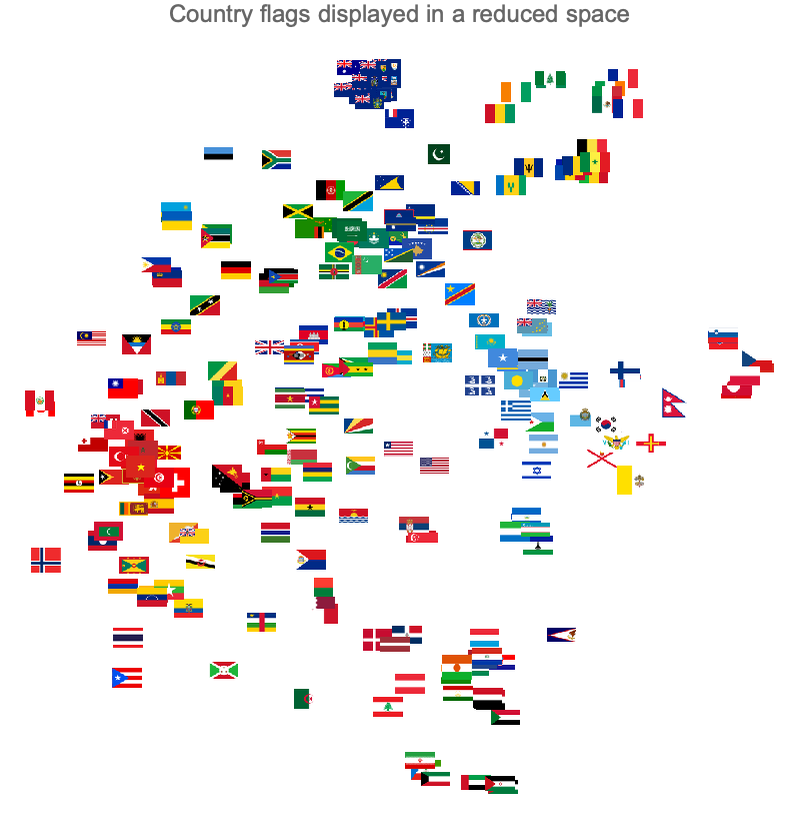Anomaly detection (see Chapter 7, Dimensionality Reduction, and Chapter 8, Distribution Learning) is another task that can be tackled in an unsupervised way. Anomaly detection concerns the identification of examples that are anomalous, a.k.a. outliers. Here is an example of anomaly detection performed on a simple numeric dataset: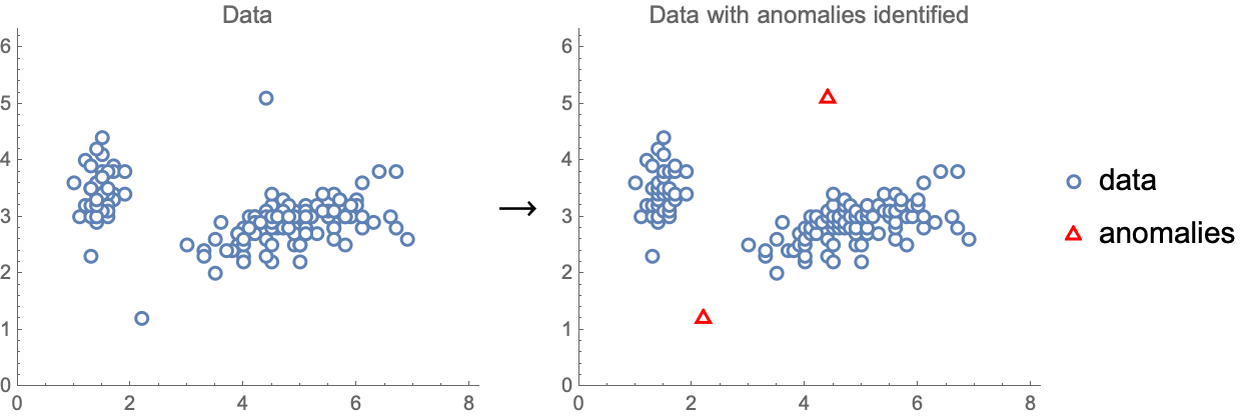This task could be useful for detecting fraudulent credit card transactions, to clean a dataset, or to detect when something is going wrong in a manufacturing process.

Another classic unsupervised task is called missing imputation (see Chapter 7 and Chapter 8), and the goal is to fill in the missing values in a dataset:This task is extremely useful because most datasets have missing values and many algorithms cannot handle them. In some cases, missing imputation techniques can also be used for predictive tasks, such as recommendation engines (see Chapter 7).

Finally, the most difficult unsupervised learning task is probably to learn how to generate examples that are similar to the training data. This task is called generative modeling (see Chapter 8) and can, for example, be used to learn how to generate new faces from many example faces. Here are such synthetic faces generated by a neural network from random noise: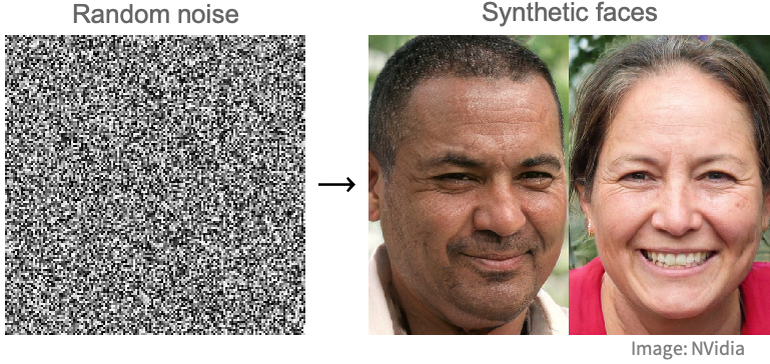Such generation techniques can also be used to enhance resolution, denoise, or impute missing values.

Unsupervised learning is a bit less used than supervised learning, mostly because the tasks it solves are less common and are harder to implement than predictive tasks. However, unsupervised learning can be applied to a more diverse set of tasks than supervised learning. Nowadays, unsupervised learning is a key element of many machine learning applications and is also used as a tool to explore data. Moreover, many researchers believe that unsupervised learning is how humans learn most of their knowledge and will, therefore, be the key to developing future artificially intelligent systems.

### Reinforcement Learning

The third most classic learning paradigm is called reinforcement learning, which is a way for autonomous agents to learn. Reinforcement learning is fundamentally different from supervised and unsupervised learning in the sense that the data is not provided as a fixed set of examples. Rather, the data to learn from is obtained by interacting with an external system called the environment. The name “reinforcement learning” originates from behavioral psychology, but it could just as well be called “interactive learning.”

Reinforcement learning is often used to teach agents, such as robots, to learn a given task. The agent learns by taking actions in the environment and receiving observations from this environment: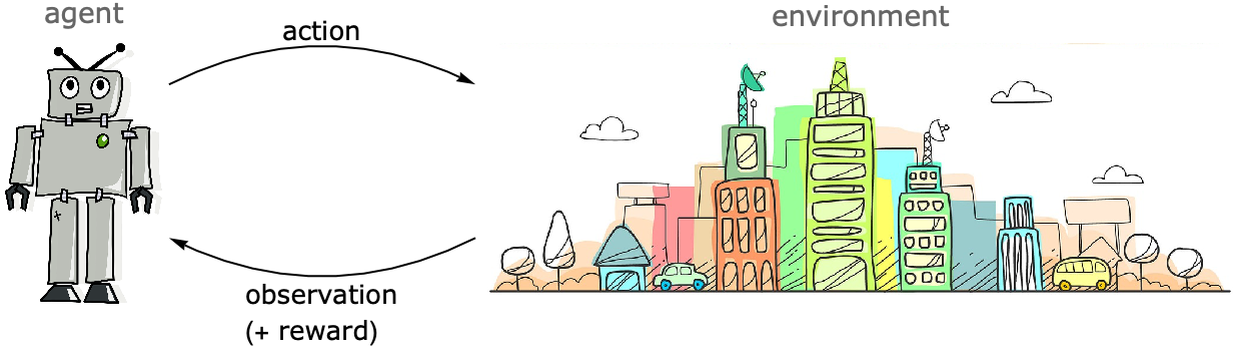Typically, the agent starts its learning process by acting randomly in the environment, and then the agent gradually learns from its experience to perform the task better using a sort of trial-and-error strategy. The learning is usually guided by a reward that is given to the agent depending on its performance. More precisely, the agent learns a policy that maximizes this reward. A policy is a model predicting which action to make given previous actions and observations.

Reinforcement learning can, for example, be used by a robot to learn how to walk in a simulated environment. Here is an snapshot from the classic Ant-v2 environment: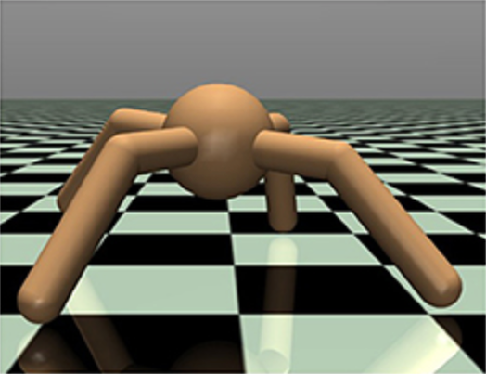In this case, the actions are the torque values applied to each leg joint; the observations are leg angles, external forces, etc.; and the reward is the speed of the robot. Learning in such a simulated environment can then be used to help a real robot walk. Such transfer from simulation to reality has, for example, been used by OpenAI to teach a robot how to manipulate a Rubik’s Cube:

It is also possible for a real robot to learn without a simulated environment, but real robots are slow compared to simulated ones and current algorithms have a hard time learning fast enough. A mitigation strategy consists of learning to simulate the real environment, a field known as model-based reinforcement learning, which is under active research.

Reinforcement learning can also be used to teach computers to play games. Famous examples include AlphaGo, which can beat any human player at the board game Go, or AlphaStar, which can do the same for the video game StarCraft: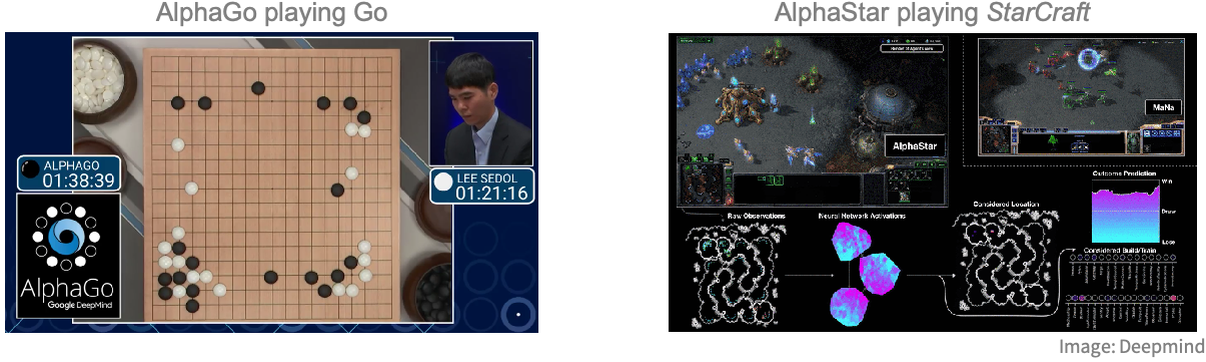Both of these programs were developed using reinforcement learning by having the agent play against itself. Note that the reward in such problems is only given at the end of the game (either you win or lose), which makes it challenging to learn which actions were responsible for the outcome.

Another important application of reinforcement learning is in the field of control engineering. The goal here is to dynamically control the behavior of a system (an engine, a building, etc.) for it to behave optimally. The prototypical example is to control a pole standing on a cart by moving the cart left or right (a.k.a. inverse pendulum):

In general, classic control methods are used for such problems, but reinforcement learning is entering this field. For example, reinforcement learning has been used to control the cooling system (fan speed, water flow, etc.) of Google data centers in a more efficient way: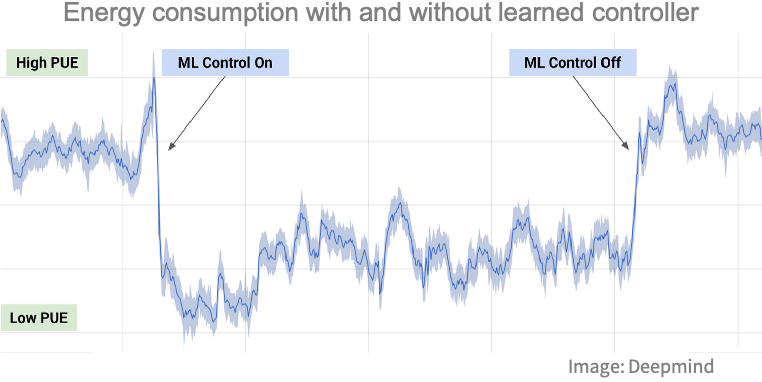One issue when applying reinforcement learning directly in such a real-world system is that during the learning phase, the agent might perform actions that can break the system or pose safety issues.

Reinforcement learning is probably the most exciting paradigm since the agent is learning by interacting, like a living being. Active systems have the potential to learn better than passive ones because they can decide by themselves what to explore in order to improve. We can imagine all sorts of applications using this paradigm, from a farmer robot that learns to improve crop production, to a program that learns to trade stocks, to a chatbot that learns by having discussions with humans. Unfortunately, current algorithms need a large amount of data to be effective, which is why most reinforcement learning applications use virtual environments. Also, reinforcement learning problems are generally more complicated to handle than supervised and unsupervised ones. For these reasons, reinforcement learning is less used than other paradigms in practical applications. As research is progressing, it is likely that algorithms will need less data to operate and that simpler tools will be developed. Reinforcement learning might then become a dominant paradigm.

Supervised, unsupervised, and reinforcement learning are the three core learning paradigms. Nevertheless, there are other ways to learn that depend on the specificities of the problem to solve. Here are a few of these other learning paradigms worth mentioning, most of which are variations or hybrids of the core paradigms.

#### Semi-supervised Learning

In semi-supervised learning, a part of the data is in the form of input-output pairs, like in supervised learning:

Another part of the data only contains inputs: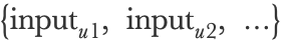The goal is generally to learn a predictive model from both of these datasets. Semi-supervised learning is thus a supervised learning problem for which some training labels are missing.

Typically, the unlabeled dataset is much bigger than the labeled dataset. One way to take advantage of this kind of data is to use a mix of unsupervised and supervised methods. Another way is to use a self-training procedure during which we train a model on the labeled data, predict the missing labels, then train on the full dataset, predict the missing labels again, and so on. Such a self-training procedure was used to obtain a state-of-the-art image identification neural network in 2019:

This network was trained with (only) 1.2 million labeled images but also with 300 million unlabeled images.

Overall, semi-supervised learning is an attractive paradigm because labeling data is often expensive. However, obtaining good results with this paradigm is a bit of an art and requires more work than supervised learning. Because of these difficulties, most machine learning users tend to stick to pure supervised approaches (which means discarding examples that do not have labels).

#### Online Learning

Online learning is a way to learn iteratively from a stream of data. In its pure form, the model updates itself after each example given: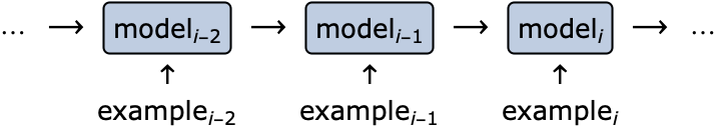The model can also update itself using batches of examples. This kind of learning could be used by a bank needing to continuously update its fraud detection system by learning from the numerous transactions made every day.

Online learning is useful when the dataset is large and comes as a stream because it avoids having to retrain models from scratch. Also, we don’t necessarily need to store the training data in this setting. Online learning is also useful because it naturally gives more importance to more recent data than to older data (which is often less relevant). Another use of online learning is when the dataset is too large to fit into the fast memory of the computer and thus needs to be read in chunks, a procedure called out-of-core learning.

Online learning is not really a paradigm in itself since the underlying problem can be both supervised (labeled examples) or unsupervised (unlabeled examples); it is more of a learning constraint. Not every machine learning method can learn online. As a rule of thumb, every method that uses a continuous optimization procedure (such as neural networks) can be used in an online learning setting.

#### Active Learning

Active learning is a way to teach a predictive model by interacting with an on-demand source of information. At the beginning of an active learning procedure, the data only consists of inputs: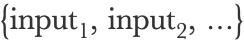During the learning procedure, the student model can request some of these unknown outputs from a teacher (a.k.a. oracle). A teacher is a system able to predict (sometimes not perfectly) the output from a given input: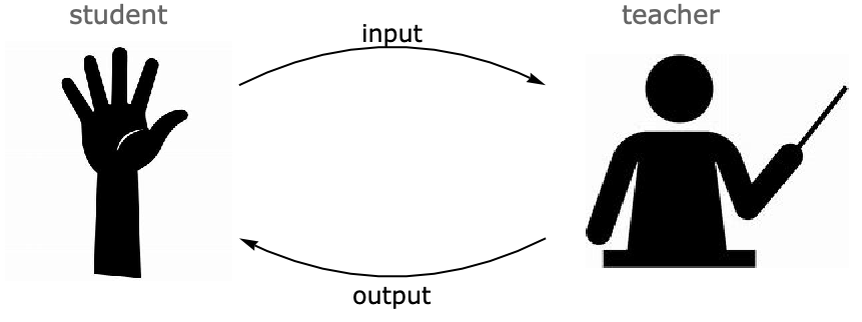Most of the time, the teacher is a human, but it could also be a program, such as a numeric simulation.

Active learning can, for example, be used to create an image classifier when training images are not labeled. In this case, humans would play the role of the teachers and the computer would decide which images should be sent for annotation.

Since the teacher is generally slow to respond, the computer must decide which example is the most informative in order to learn as fast as possible. For example, it might be smart to ask the teacher about inputs that the model cannot predict confidently yet.

Active learning can be seen as a subset of reinforcement learning since the student is also an active agent. The difference is that the agent cannot alter the environment here. Such active systems have the potential to learn much faster than passive systems, and this might be a key to creating intelligent systems.

#### Transfer Learning

Transfer learning deals with transferring knowledge from one learning task to another learning task. It is typically used to learn more efficiently from small datasets when we have access to a much larger dataset that is similar (but different). The strategy is generally to train a model on the large dataset and then use this pre-trained model to help train another model on the task that we really care about: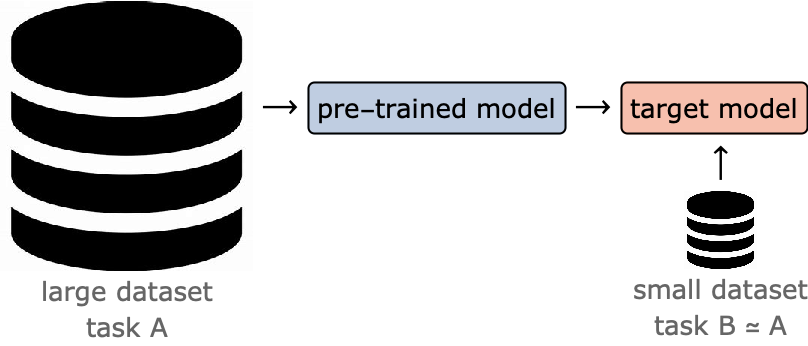Let’s use a transfer learning procedure to train a new mushroom classifier on the same 16 examples used in the first chapter:

Identifying images from scratch requires many more training examples. For example, the neural network behind the ImageIdentify function has been trained on about 10 million images:

This model can distinguish between about 4000 objects, but it is not detailed enough for our task:

It is possible to adapt it to our task though. This network has 24 layers that gradually improve the understanding of the image (see Chapter 11, Deep Learning Methods). In a nutshell, the first layers identify simple things, such as lines and simple shapes, while the last layers can recognize high-level concepts (although not necessarily human-understandable concepts such as “cap color” or “gills type”). We are going to use the first 22 layers of this network as a feature extractor. This means that we are going to preprocess each image with a truncated network to obtain features that are semantically richer than pixel values. We can then train a classifier on top of these new features:

The classifier can now recognize our mushrooms:

This classifier obtains about 85% accuracy on a test set constructed from a web image search:

This is not perfect, but if we were to train directly on the underlying pixel values, we would obtain about 50% accuracy, which is no better than random guessing:

This is a simple example of transfer learning. We used a network trained on a large dataset in order to extract a useful vector representation (a.k.a. latent features) for our related downstream task. There are other transfer learning techniques that are similar in spirit, and they generally also involve neural networks.

Transfer learning is heavily used to learn from image, audio, and text data. Without transfer learning, it would be hard to accomplish something useful in these domains. Transfer learning is not used much on typical structured data however (bank transactions, sales data, etc.). The reason for that is that structured datasets are somewhat unique, so it is harder to transfer knowledge from one to another. That might not always stay this way in the future; after all, our brains are doing some kind of transfer learning all the time, reusing already-learned concepts in order to learn new things faster.

#### Self-Supervised Learning

Self-supervised learning generally refers to a supervised learning problem for which the inputs and outputs can be obtained from the data itself, without needing any human annotation. For example, let’s say that we want to predict the next word after a given sequence of English words. To learn how to do this, we can use a dataset of sentences: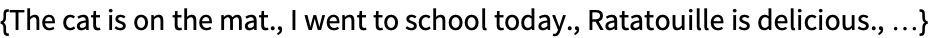We can then transform this dataset into a supervised learning problem: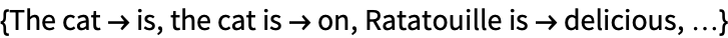The input-output pairs are therefore obtained from the data itself. As another example, let’s say we want to learn how to colorize images. We can take images that are already in color and convert them to grayscale to obtain a supervised dataset:

Again, the prediction task is already present in the data. There are plenty of other applications like this (predicting missing pixel values, predicting the next frame from a video, etc.).

Self-supervised learning is not really a learning paradigm since it refers to how the data was obtained, but it is a useful term to represent this class of problems for which labeling is free. Typically, self-supervised learning is used to learn a representation (see Chapter 7, Dimensionality Reduction), which is then used to tackle a downstream task through a transfer learning procedure. The self-supervised task is then called the pretext task or auxiliary task. Both next-word prediction and image colorization are examples of such pretext tasks that are used for transfer learning.

Vocabulary
Supervised Learning
 unsupervised learning learning from data examples that do not have labels clustering separating data examples into groups dimensionality reduction reducing the number of variables in a dataset while preserving some properties of the data anomaly detection identifying examples that are anomalous anomalyoutlier data example that substantially differs from other data examples imputation filling in missing values of a dataset generative modeling learning to generate synthetic data examples
Reinforcement Learning
 reinforcement learning learning by interacting with an environment environment external system that the reinforcement learning agent interacts with actions things that the agent does in the environment observations feedback given by the environment reward special observation given by the environment to inform the agent if the task is well done policy model predicting which action to make given previous actions and observations model-based reinforcement learning reinforcement learning where a model is trained to simulate the real environment
Exercises
2.1Find which paradigm can be used to tackle the applications described in Chapter 1.
Tech Notes
Unsupervised vs. Supervised

In this book, we define supervised learning as learning from input-output pairs and unsupervised learning as learning from unlabeled data. This distinction only concerns the form of the data and not the type of data (image, text, etc.) or the method used. Researchers and expert practitioners can have a slightly different (and more fuzzy) definition, which is more related to which method is used to solve the task. As an example, imagine the goal is to generate images given their class using a dataset such as:

Technically, this is a supervised problem, but many would call it unsupervised because the labels have many degrees of freedom (the pixels), which means that the methods used to tackle such a task are very similar to the methods used in a pure unsupervised setting (such as learning the distribution of images without classes). Both definitions are useful depending on the context.